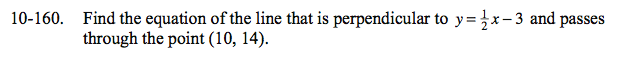Home > CCA2 > Chapter 10 > Lesson 10.3.1 > Problem10-160

10-160.

Find the equation of the line that is perpendicular to y =x − 3 and passes through the point (10, 14). Homework Help ✎What is the slope of the perpendicular line?
Recall thatperpendicular slopes have a product of −1.

If you use y = mx + b, use the given (x, y) point to solve for b.

y = −2x + b
14 = −2(10) + b